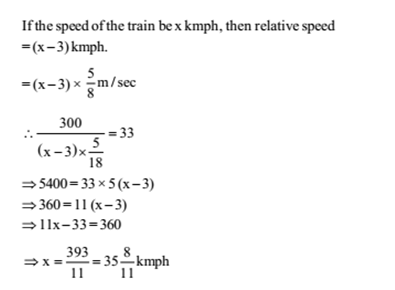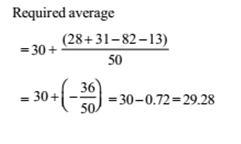# RRB ALP 2018 Practice Test Papers | Arithmetic Questions (Day-35)

Dear Aspirants, Here we have given the Important RRB ALP & Technicians Exam 2018 Practice Test Papers. Candidates those who are preparing for RRB ALP 2018 can practice these Arithmetic Questions to get more confidence to Crack RRB 2018 Examination.

[WpProQuiz 2084]

Click “Start Quiz” to attend these Questions and view Explanation

1. If 60% of A = 3 /4 of B, then A: B is

(a) 9: 20

(b) 20: 9

(c) 4: 5

(d) 5: 4

1. A shopkeeper earns a profit of 12% on selling a book at 10% discount on the printed price. The ratio fo the cost price and the printed price of the book is

(a) 45: 56

(b) 45: 51

(c) 47: 56

(d) 47: 51

1. A train, 300 m long, passed a man, walking along the line in the same direction at the rate of 3 km/hr in 33 seconds. The speed of the train is

(a) 30 km/hr

(b) 32 km/hr

(c) 32 8/11 km/hr

(d) 35 8/11 km/hr

1. If the circumference of a circle is decreased by 50% then the percentage of decrease in its area is

(a) 25

(b) 50

(c) 60

(d) 75

1. What must be added to each term of the ratio 7: 11, so as to make it equal to 3: 4?

(a) 8

(b) 7.5

(c) 5

(d) 6. 5

1. The line passing through (–2, 5) and (6,b) is perpendicular to the line 20x + 5y = 3. Find b?

(a) –7

(b) 4

(c) 7

(d) –4

1. In an examination, a student scores 4 marks for every correct answer and loses 1 mark for every wrong answer. A student attempted all the 200 questions and scored, in all 200 marks. The number of questions, he answered correctly was

(a) 82

(b) 80

(c) 68

(d) 60

1. The mean of 50 numbers is 30. Later it was discovered that two entries were wrongly entered as 82 and 13 instead of 28 and 31. Find the correct mean.

(a) 29.28

(b) 30.66

(c) 38.12

(d) 38.21

1. The unit’s digit in the product 735x 371x1155 is:

(a) 1

(b) 3

(c) 7

(d) 9

1. A number, when divided by 136, leaves remainder 36. If the same number is divided by 17, the remainder will be

(a) 9

(b) 7

(c) 3

(d) 2

A x60 /100=3B/4

A/B= 5/4

Let the CP be Rs.100.

SP = Rs.112

If the marked price be Rs.x, then 90% of x = 112

112x 100 x/90   = Rs. 1120/ 9

Required ratio 100: 1120 /9 = = 900: 1120 = 45: 56

1. Answer: (d)Circumference = 2pi r

The decrease in area = – 50 – 50 + 50x 50/ 100 = –75%

Let the required number be x.( 7+x )/(11 +x )=3/4

28 + 4x = 33 + 3x

=>x = 33 – 28 = 5

Here, 20x + 5y = 3 => 5y = – 20x + 3

y = – 4x + 3/ 5

Slope of 20x + 5y = 3 => –4

We know, product of slopes = –1 for perpendicular lines

Hence, the slope of the line which passes through (–2, 5) and (6, b) =(b-5)/(6-(-2))

Now, =( b-5)/(6+2)= 1/4 => b – 5 = 2

b = 5 + 2 = 7

If the number of correct answers be x, then x × 4 – 1. (200 – x) = 200

=> 4x – 200 + x = 200

=> 5x = 400 => x = 400 5 = 80

1. Answer: (a)Unit digit in 74 and34 =1

Therefore Unit digit in735 =Unit digit in( 732x 73) = 1x7x7x7 =3

Unit digit in371=Unit digit in (368 x 33) =1x3x3x3=7

unit’s digit in the product 735 x 371x 1155=3x7x1=1

If the first divisor be a multiple of the second divisor, then required remainder = remainder obtained by dividing the first remainder (36) by the second divisor (17) = 2

17 is a factor of 136

Remainder when 36 is divided by 17 = 2

RRB ALP 2018 Practice Test Papers | Arithmetic Questions (Day-1)

RRB ALP 2018 Practice Test Papers | Arithmetic Questions (Day-2)

RRB ALP 2018 Practice Test Papers | Arithmetic Questions (Day-3)

RRB ALP 2018 Practice Test Papers | Arithmetic Questions (Day-4)

RRB ALP 2018 Practice Test Papers | Arithmetic Questions (Day-5)

RRB ALP 2018 Practice Test Papers | Arithmetic Questions (Day-6)

RRB ALP 2018 Practice Test Papers | Arithmetic Questions (Day-7)

RRB ALP 2018 Practice Test Papers | Arithmetic Questions (Day-8)

RRB ALP 2018 Practice Test Papers | Arithmetic Questions (Day-9)

RRB ALP 2018 Practice Test Papers | Arithmetic Questions (Day-10)

RRB ALP 2018 Practice Test Papers | Arithmetic Questions (Day-11)

RRB ALP 2018 Practice Test Papers | Arithmetic Questions (Day-12)

RRB ALP 2018 Practice Test Papers | Arithmetic Questions (Day-13)

RRB ALP 2018 Practice Test Papers | Arithmetic Questions (Day-14)

RRB ALP 2018 Practice Test Papers | Arithmetic Questions (Day-15)

RRB ALP 2018 Practice Test Papers | Arithmetic Questions (Day-16)

RRB ALP 2018 Practice Test Papers | Arithmetic Questions (Day-17)

RRB ALP 2018 Practice Test Papers | Arithmetic Questions (Day-18)

RRB ALP 2018 Practice Test Papers | Arithmetic Questions (Day-19)

RRB ALP 2018 Practice Test Papers | Arithmetic Questions (Day-20)

RRB ALP 2018 Practice Test Papers | Arithmetic Questions (Day-21)

RRB ALP 2018 Practice Test Papers | Arithmetic Questions (Day-22)

RRB ALP 2018 Practice Test Papers | Arithmetic Questions (Day-23)

RRB ALP 2018 Practice Test Papers | Arithmetic Questions (Day-24)

RRB ALP 2018 Practice Test Papers | Arithmetic Questions (Day-25)

RRB ALP 2018 Practice Test Papers | Arithmetic Questions (Day-26)

RRB ALP 2018 Practice Test Papers | Arithmetic Questions (Day-27)

RRB ALP 2018 Practice Test Papers | Arithmetic Questions (Day-28)

RRB ALP 2018 Practice Test Papers | Arithmetic Questions (Day-29)

RRB ALP 2018 Practice Test Papers | Arithmetic Questions (Day-30)

RRB ALP 2018 Practice Test Papers | Arithmetic Questions (Day-31)

RRB ALP 2018 Practice Test Papers | Arithmetic Questions (Day-32)

RRB ALP 2018 Practice Test Papers | Arithmetic Questions (Day-33)

RRB ALP 2018 Practice Test Papers | Arithmetic Questions (Day-34)